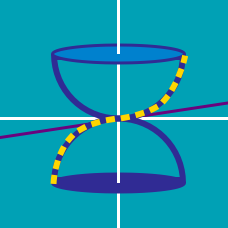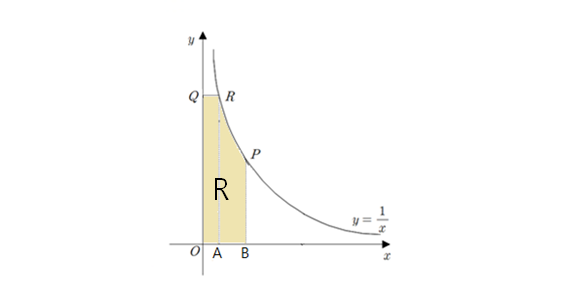Calculus

# Volume of Revolution - Shell MethodThe above diagram shows the points $R=(7,\frac{1}{7})$ and $P=(14,\frac{1}{14}),$ which lie on the curve $y=\frac{1}{x},$ point $Q = (0, \frac{1}{7})$ on the $y$-axis, and points $A = (7,0)$ and $B = (14,0)$ on the $x$-axis. If $S$ is the solid obtained by rotating the region $R$ bounded by $\overline{OQ}, \overline{QR}, \widehat{RP}, \overline{PB}, \text{ and } \overline{OB}$ about the axis of rotation $x=-7$ and the volume of $S$ is $\alpha \pi,$ what is $\alpha?$

Note: The above diagram is not drawn to scale.

Let $S$ be the solid obtained by rotating the region $R$ bounded by $y = x^2$ and $y = \sqrt{x}$ about the line $x=-10$. If the volume of $S$ is $\alpha \pi,$ what is $\alpha?$

Let $S$ be the solid obtained by rotating the region $R$ bounded by $y = x-9, x = 15,$ and the $x$-axis about the axis of rotation $x=19 .$ If the volume of $S$ is $\alpha \pi,$ what is $\alpha?$

Let $S$ be the solid obtained by rotating the region $R$ bounded by $y = x^3, x = 1, x = 5,$ and the $x$-axis about the line $x=8$. If $10$ times the volume of $S$ is $\alpha \pi ,$ what is $\alpha?$

Let $R$ be the region bounded by $y = 3x+2 , x = 4$, and the x-axis. Let $S$ be the solid obtained by rotating $R$ about the axis $x=0$. The volume of $S$ has the form $T \pi$. What is the value of $T$?

×